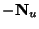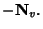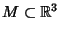## Shape Operator

The negative derivative(1)

of the unit normalvector field of a Surface is called the shape operator (or Weingarten Map or Second Fundamental Tensor). The shape operatoris an Extrinsic Curvature, and the Gaussian Curvature is given by the Determinant of. Ifis a Regular Patch, then(2)(3)

At each point p on a Regular Surface, the shape operator is a linear map(4)

The shape operator for a surface is given by the Weingarten Equations.

Gray, A. The Shape Operator,'' Calculation of the Shape Operator,'' and The Eigenvalues of the Shape Operator.'' §14.1, 14.3, and 14.4 in Modern Differential Geometry of Curves and Surfaces. Boca Raton, FL: CRC Press, pp. 268-269, 274-279, 1993.